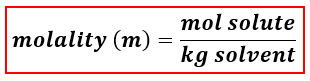# Problem: Calculate the molality of total ions in an aqueous solution prepared by dissolving 0.400 moles of magnesium chloride in 850.0 g of water.A. 0.47 x 10-4 mB. 1.41 x 10-4 mC. 1.41 mD. 0.47 mE. 0.941 m

###### FREE Expert Solution
91% (424 ratings)
###### FREE Expert Solution

We’re being asked to calculate for the molality of total ions in an aqueous solution prepared by dissolving 0.400 moles of magnesium chloride in 850.0 g of water.

When calculating for molality, we use the following equation:The moles of MgCl2 is given but we were asked to determine the molality of total ions

We will calculate the molality of the solution using the following steps:

Step 1. Determine the ions present in the solution.
Step 2. Calculate the moles of the ions present in the solution.
Step 3. Calculate the mass of the solvent (in kg).
Step 4. Calculate the molality of total ions.

Step 1. Determine the ions present in the solution.

91% (424 ratings)###### Problem Details

Calculate the molality of total ions in an aqueous solution prepared by dissolving 0.400 moles of magnesium chloride in 850.0 g of water.

A. 0.47 x 10-4 m

B. 1.41 x 10-4 m

C. 1.41 m

D. 0.47 m

E. 0.941 m

What scientific concept do you need to know in order to solve this problem?

Our tutors have indicated that to solve this problem you will need to apply the Molality concept. You can view video lessons to learn Molality. Or if you need more Molality practice, you can also practice Molality practice problems.

What is the difficulty of this problem?

Our tutors rated the difficulty ofCalculate the molality of total ions in an aqueous solution ...as medium difficulty.

How long does this problem take to solve?

Our expert Chemistry tutor, Sabrina took 1 minute and 42 seconds to solve this problem. You can follow their steps in the video explanation above.

What professor is this problem relevant for?

Based on our data, we think this problem is relevant for Professor Bates' class at APP STATE.